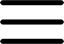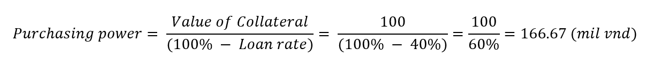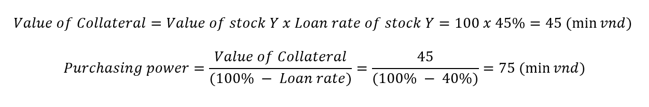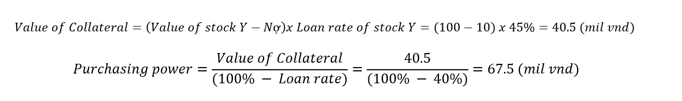Concept: Margin buying power is the maximum amount a customer can buy securities on a margin account at each transaction time. Margin buying power is calculated by Pinetree based on client assets including money, stocks and debt.

Example 1

You have 100 million in cash in your margin account and intend to buy stock X on the margin account. Currently, Stock X is in Pinetree’s margin portfolio with a 40% loan ratio. At that time, the collateral used to buy securities is VND 100 million in cash, your margin purchasing power is calculated as follows:Example 2

You own Stock Y with a total value of VND 100 million on a margin account and have no debt yet, the current lending rate for stock Y is 45%. You want to buy stock X with a loan rate of 40%.

Then:Example 3:

You are owning stock Y with a total value of VND 100 million on a margin account, of which 90 million is equity and 10 million borrowed from Pinetree, the current lending rate of stock Y is 45%. You want to buy stock X with a loan rate of 40%.

Then: